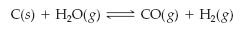×
Get Full Access to Introductory Chemistry - 5 Edition - Chapter 15 - Problem 65p
Get Full Access to Introductory Chemistry - 5 Edition - Chapter 15 - Problem 65p

×

# Answer: Consider this reaction at equilibrium. Predict the effect (shift right, shiftISBN: 9780321910295 34

## Solution for problem 65P Chapter 15

Introductory Chemistry | 5th Edition

• Textbook Solutions
• 2901 Step-by-step solutions solved by professors and subject experts
• Get 24/7 help from StudySoup virtual teaching assistantsIntroductory Chemistry | 5th Edition

4 5 1 402 Reviews
30
5
Problem 65P

Problem 65P

Consider this reaction at equilibrium.Predict the effect (shift right, shift left, or no effect) of these changes.

(a) adding C to the reaction mixture

(b) condensing H2O and removing it from the reaction mixture

(c) adding CO to the reaction mixture

(d) removing H2 from the reaction mixture

Step-by-Step Solution:

Solution:

Step 1

From  this given equilibrium  reactionWe have to predict the effect (shift right, shift left, or no effect) of these changes.

(a) adding C to the reaction mixture

(b) condensing H2O and removing it from the reaction mixture

(c) adding CO to the reaction mixture

(d) removing H2 from the reaction mixture

Step 2 of 3

Step 3 of 3

##### ISBN: 9780321910295

Unlock Textbook Solution